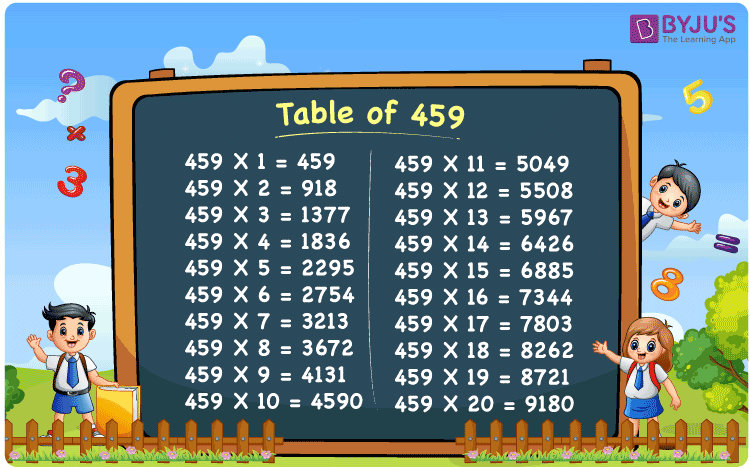Checkout JEE MAINS 2022 Question Paper Analysis : Checkout JEE MAINS 2022 Question Paper Analysis :

# Table of 459

The table of 459 is also known as the 459 times table and is a multiplication table that results in the product of 459 with the consecutive natural numbers. The 459 times table represents the repetitive addition of the number 459. For example, multiplying 459 by 2 is equivalent of adding 459 two times (459 × 2 = 459 + 459 = 918). To solve tricky multiplication problems that include multiples of 459, students must memorize the table of 459.

## Table of 459 Chart## What is the 459 Times Table?

As we know, math tables can be written in a variety of ways, including repeated addition. We can create the 459 times table either by addition or multiplication. The table below shows the 459 times table up to 10 results.

 Using multiplication Using repeated addition 459 × 1 = 459 459 459 × 2 = 918 459 + 459 = 918 459 × 3 = 1377 459 + 459 + 459 = 1377 459 × 4 = 1836 459 + 459 + 459 + 459 = 1836 459 × 5 = 2295 459 + 459 + 459 + 459 + 459 = 2295 459 × 6 = 2754 459 + 459 + 459 + 459 + 459 + 459 = 2754 459 × 7 = 3213 459 + 459 + 459 + 459 + 459 + 459 + 459 = 3213 459 × 8 = 3672 459 + 459 + 459 + 459 + 459 + 459 + 459 + 459 = 3672 459 × 9 = 4131 459 + 459 + 459 + 459 + 459 + 459 + 459 + 459 + 459 = 4131 459 × 10 = 4590 459 + 459 + 459 + 459 + 459 + 459 + 459 + 459 + 459 + 459 = 4590

## Multiplication Table of 459

Go through the multiplication table of 459 given here, up to 20 times.

 459 × 1 = 459 459 × 2 = 918 459 × 3 = 1377 459 × 4 = 1836 459 × 5 = 2295 459 × 6 = 2754 459 × 7 = 3213 459 × 8 = 3672 459 × 9 = 4131 459 × 10 = 4590 459 × 11 = 5049 459 × 12 = 5508 459 × 13 = 5967 459 × 14 = 6426 459 × 15 = 6885 459 × 16 = 7344 459 × 17 = 7803 459 × 18 = 8262 459 × 19 = 8721 459 × 20 = 9180

## Example on the Table of 459

Question:

Michael bakes 15 cakes in a day. In 459 days, how many cakes will he bake?

Solution:

Given that Michael bakes 15 cakes in one day.

Number of days = 459

The total number of cakes baked by Michael in 459 days = 459 × 15 = 6885.

## Frequently Asked Questions on Table of 459

### What is the table of 459?

The table of 459 is the multiplication of 459 by 1, 2, 3, and so on. This can be written as 459 × 1 = 459, 459 × 2 = 918, 459 × 3 = 1377, 459 × 4 = 1836, 459 × 5 = 2295, etc.

### What should be multiplied by 459 to get 5508?

459 should be multiplied by 12 to get 5508 since 459 times 12 is 5508, i.e. 459 × 12 = 5508.

### How do you write the value of 459 times 10?

We can write the value of 459 times 10 in two different ways as given below.
Using multiplication: 459 times 10 = 459 × 10 = 4590
Using repeated addition: 459 times 10 = 459 + 459 + 459 + 459 + 459 + 459 + 459 + 459 + 459 + 459 = 4590